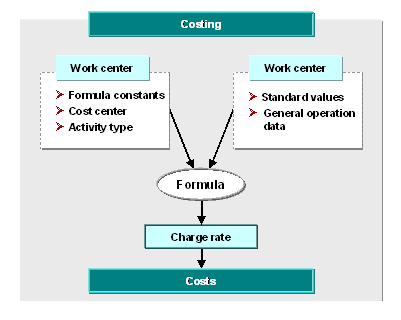Show TOC

###Setting up a Quantity StructureThe most important data for setting up a quantity structure for an activity is defined in the work center:

• Activity types

• Formulas

Every activity which is calculated is allocated to an activity type. A formula is allocated to each activity type in the work center. Using the formula and the standard times in the operation, the system calculates how much activity is expected for a particular activity type in a particular operation.

As soon as the system has calculated how much activity is expected for a particular activity type, it can valuate the activity per activity type.( )